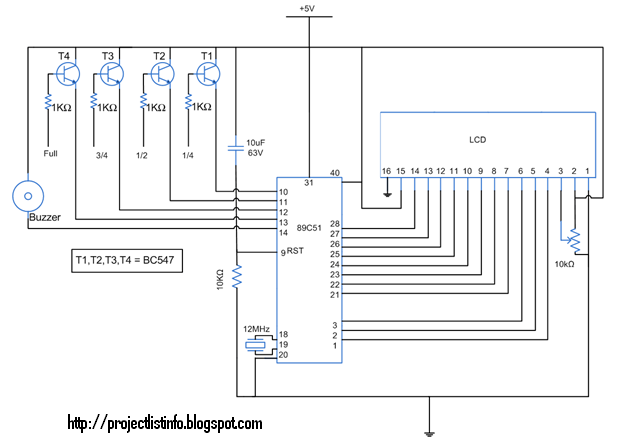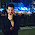## Pages

### Liquid level alarm using 8051 microcontroller (AT89C51)This article illustrates the construction and working of a liquid level indicator. Such an indicator is used in tanks to indicate the level of liquids and alert us when the tank is full. So by this circuit we can monitor the various levels of the tank and can avoid spillage of water and also we can configure our supplies according to the various levels of tank. Such module or circuit can be installed in big buildings where manual monitor of tanks is difficult and its indicator can be placed at some centralised place.
This circuit works on the principle that water conducts electricity. A wire connected to VCC and four other wires are dipped in tank at different levels namely quarter, half, three-fourth, full and their output are taken on pins P3.0, P3.1, P3.2, P3.3 via a transistor BC547. Port P2 is connected to data pins of LCD and P1.0, P1.1, P1.2 are respectively connected to RS, RW, and EN pins of LCD.
Initially when the tank is empty LCD will show the message VACANT. As the tank starts filling up wire at different levels get some positive voltage, due to conducting nature of water. This voltage is then fed to their corresponding pins on controller. When level reaches to quarter level, LCD displays the message QUARTER. On further rise of level, HALF and 3/4 QUARTER are displayed on LCD. When tank gets full LCD shows the message FULL CLOSE TAP. A buzzer is also provided to produce a alert the user when the tank gets filled. This buzzer can be made off by pressing the switch connected between pin 15 of controller and VCC.
C Code:
// Program to make a Liquid level indicator using LCD
#include<reg51.h>
sbit rs=P1^0; //register select pin
sbit rw=P1^1; //read/write pin
sbit e=P1^2; //enable pin
sbit quat=P3^0; //pin connected to quater level of tank
sbit half=P3^1; //pin connected to half level of tank
sbit quat_3=P3^2; //pin connected to three -fourth level of tank
sbit full=P3^3; //pin connected to full level of tank
sbit spkr_on=P3^4;
sbit spkr_off=P3^5; // pin to off speaker

void delay(int k) //delay function
{
int i,j;
for(i=0;i<k;i++)
for(j=0;j<1275;j++);
}

void write(int j) //write function
{
rs=1;  //selecting command register
rw=0;  //selecting to write
P2=j;  //putting value on the pins
e=1;  //strobe the enable pin
delay(1);
e=0;
return;
}

void cmd(int j)  //command function
{
P2=j;  //put the value on pins
rs=0;  //selecting command register
rw=0;  //selecting to write
e=1;  //strobe enable pin
delay(1);
e=0;
return;
}

void puts(char *a) //puts function to print a string
{
unsigned int p=0;
for(;a[p]!=0;p++)
write(a[p]);
}

void lcd_init(void) // function to initialise the LCD
{
cmd(0x38); //setting 8-bit interface, 2 lines, 5*7 Pixels
delay(1);
cmd(0x0e); //turning on underline visible cursor
delay(1);
cmd(0x01); //clearing screen
cmd(0x80); //moving cursor to the begining of line 1 of LCD
}

void main()
{
quat=half=quat_3=full=spkr_off=1; //configuring as input pins
quat=half=quat_3=full=spkr_off=0; //lowering input pins
spkr_on=1;    // making speaker on pin high,as it works on negative logic
while(1)
{
while(quat==0&&half==0&&quat_3==0&&full==0&&spkr_off==0)   //condition when tank is empty
{
lcd_init();        // initialising LCD
puts("VACANT");       //printing VACANT on lcd
}
while(quat==1&&half==0&&quat_3==0&&full==0&&spkr_off==0)        //condition when tank is quater
{
lcd_init();
puts("QUATER");      //printing QUATER on lcd
}
while(quat==1&&half==1&&quat_3==0&&full==0&&spkr_off==0)        //condition when tank is half
{
lcd_init();
puts("HALF");      //printing HALF on lcd
}
while(quat==1&&half==1&&quat_3==1&&full==0&&spkr_off==0)        //condition when tank is three-fourth
{
lcd_init();
puts("3/4 FULL");     //printing 3/4 FULL on lcd
}
while(quat==1&&half==1&&quat_3==1&&full==1&&spkr_off==0)        //condition when tank is full
{
lcd_init();
puts("FULL;CLOSE TAP");     //printing FULL;CLOSE TAP on lcd
spkr_on=0;// Enabling speaker
}
while(quat==1&&half==1&&quat_3==1&&full==1&&spkr_on==0&&spkr_off==1)//enabling high speaker_off pin
{
spkr_on=1;//disabling speaker
}

}

1.hey karan..
i would like to have the codes for the above ckt..
karachheda24593@gmail.com

2.i want code plzzzzz with full descriptionn urgently plzz zain.raza24@gmailcom

3.i need code with full description plzzzz mail meee urgently needed zain.raza24 @gmail.com

4.wahm1234@hotmail.com

IF you any query about any project related than write your comment in comment box